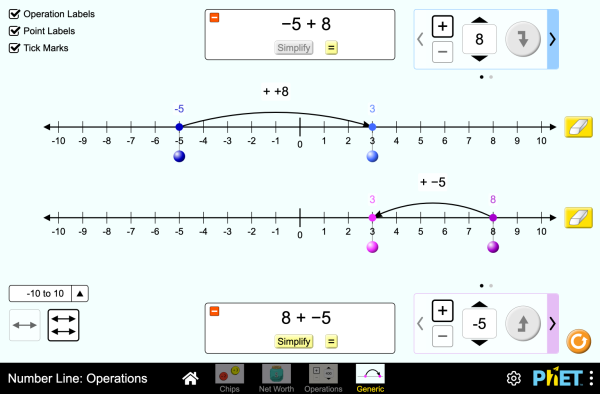# Number Line: Operations

این شبیه سازی هنوز به این زبان ترجمه نشده است. شما می توانید نسخه ی انگلیسی را ببینید.دانلودEmbed کردن ببند نسخه فعلی این شبیه سازی را embed کنید از این فایل HTML برای embed کردن نسخه فعلی این شبیه سازی استفاده کنید. ضمنا میتوانید طول و عرض را عوض کنید. تصویری را embed کنید که با کلیک کردن روی آن شبیه سازی اجرا شود.
برای اجرا کلیک کنید
از این کد برای اجرای تصویری که پیغام میدهد "برای اجرا اینجا را کلیک کنید" استفاده کنید. Operations Number Line Integers PhET حمایت می شود توسطو مدرسانی چون شما.

• Operations
• Number Line
• Integers

### Description

Explore operations with integers using an authentic context of net worth, then generalize with a number line. Experiment with adding and subtracting both positives and negatives, and make predictions about whether the total will be positive or negative.

### نمونه ای از هدف های آموزشی

• Represent addition and subtraction of integers on a horizontal number line
• Reason about addition and subtraction of integers in terms of number-locations
• Use logical necessity to reason that addition has the opposite effect as subtraction or that adding (or subtracting) a negative integer has the opposite effect as adding (or subtracting) a positive integer.
• Recognize and generate equivalence classes of integer sums and differences
• Show that a number and its additive inverse (opposite) have a sum of 0
• Using a net worth context, describe situations that have a positive sum or difference, negative sum or difference, and zero sum or difference
• View adding a negative as equivalent to subtracting a positive, and explain why this relationship makes sense in contexts such as net worth.
• View subtracting a negative as equivalent to adding a positive, and explain why this relationship makes sense in contexts such as net worth.
• Apply a number line model for addition and subtraction to new contexts.

### Standards Alignment

#### Common Core - Math

7.NS.A.1
Apply and extend previous understandings of addition and subtraction to add and subtract rational numbers; represent addition and subtraction on a horizontal or vertical number line diagram.
7.NS.A.1a
Describe situations in which opposite quantities combine to make 0. For example, a hydrogen atom has 0 charge because its two constituents are oppositely charged.
7.NS.A.1b
Understand p + q as the number located a distance |q| from p, in the positive or negative direction depending on whether q is positive or negative. Show that a number and its opposite have a sum of 0 (are additive inverses). Interpret sums of rational numbers by describing real-world contexts.
7.NS.A.1c
Understand subtraction of rational numbers as adding the additive inverse, p - q = p + (-q). Show that the distance between two rational numbers on the number line is the absolute value of their difference, and apply this principle in real-world contexts.
7.NS.A.1d
Apply properties of operations as strategies to add and subtract rational numbers.
Version 1.0.1

### Teacher Tipsراهنمای معلم مجموعه توصیه های تولید شده توسط گروه PHET به صورت pdf. ( PDF ).

### Teacher-Submitted Activities

Integer Addition and Subtraction Assessment Gillian Walker دبیرستان
راهنمایی
آزمایشگاه
Remote
کار در خانه
ریاضیات
آلمانی All DeutschZahlenstrahl: Operationen
اسلواکی All SlovenčinaČíselná os: Operácie
اسپانیایی All españolRecta Numérica: Operacionesاسپانیایی، پرو All español (Perú)Recta numérica: Operaciones
ایتالیایی All italianoRetta dei numeri: operazioni
باسک All EuskaraZenbaki-lerroa: eragiketak
بوسنیایی All BosanskiBrojevna prava: Operacije
ترکی All TürkçeSayı Doğrusu: İşlemler
دانمارکی All DanskTallinje: Beregninger
صرب All СрпскиБројевна права: Операције
فرانسوی All françaisDroite numérique: opérations
قبایل مائوري زلاند جدید All MaoriRārangi Tau: Paheko
لتونی All LatviešuSkaitļu ass: Darbības
لهستانی All polskiOś liczbowa: działania
مجاری All magyarSzámegyenes - műveletek
هلندی All NederlandsGetallijn: Bewerkingen
ویتنامی All Tiếng ViệtĐường thẳng số: Các phép toán
پرتغالی، برزیلی All português (Brasil)Reta Numérica: Operações
چینی، قدیمی All 中文 (台灣)Number Line: Operations_數線：數線的運算
کرواتی All hrvatskiBrojevna crta: Računske operacije
یونانی All ΕλληνικάΑριθμογραμμή: πράξεις

HTML5 sims can run on iPads and Chromebooks, as well as PC, Mac, and Linux systems on modern web browsers. If you are experiencing issues using an HTML5 sim on a supported platform, please disable any browser extensions.

iOS 12+ Safari

Android:
Not officially supported. If you are using the HTML5 sims on Android, we recommend using the latest version of Google Chrome.

Chromebook:
The HTML5 and Flash PhET sims are supported on all Chromebooks.
Chromebook compatible sims

Windows Systems: